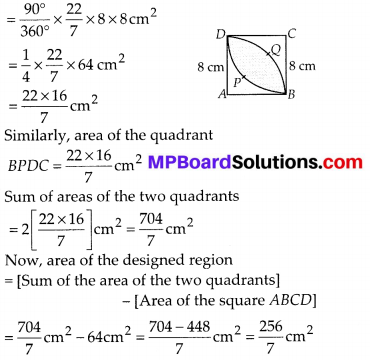In this article, we will share MP Board Class 10th Maths Book Solutions Chapter 12 Areas Related to Circles Ex 12.3 Pdf, These solutions are solved subject experts from the latest edition books.

## MP Board Class 10th Maths Solutions Chapter 12 Areas Related to Circles Ex 12.3

Unless stated otherwise, use π = $$\frac{22}{7}$$

Question 1.
Find the area of the shaded region in the given figure, if PQ = 24 cm, PR = 7cm and O is the centre of the circle.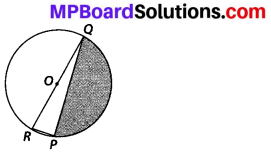Solution:
Since O is the centre of the circle,
∴ QOR is a diameter.
⇒ ∠RPQ = 90° [Angle in a semicircle]
Now, in right ∆RPQ, RQ2 = PQ2+ PR2 [Pythagoras theorem]
⇒ RQ2 = 242 + 72 = 576 + 49 = 625
⇒ RQ = $$\sqrt{625}$$ = 25 cm
∴ Radius of a circle (r) = $$\frac{25}{2}$$ cm
∴ Area of ∆RPQ
= $$\frac{1}{2}$$ × PQ × RP = $$\frac{1}{2}$$ × 24 × 7cm2
= 12 × 7 cm2 = 84 cm2
Now, area of semicircle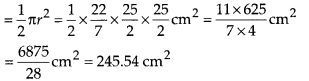∴ Area of the shaded portion
= 245.54 cm2 – 84 cm2 = 161.54 cm2

Question 2.
Find the area of the shaded region in given figure, if radii of the two concentric circles with centre O are 7 cm and 14 cm respectively and ∠AOC = 40°.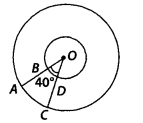Solution:
Radius of the outer circle (R) = 14 cm and θ = 40°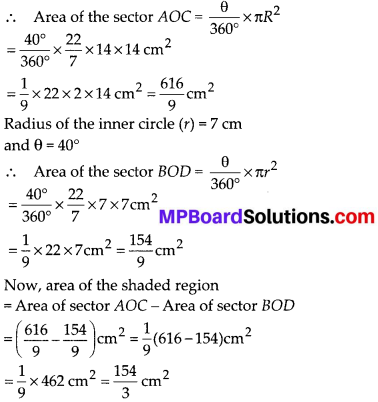Question 3.
Find the area of the shaded region in the given figure, if ABCD is a square of side 14 cm and APD and BPC are semicircles.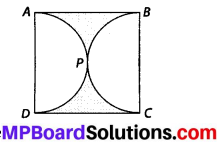Solution:
Side of the square = 14 cm
∴ Area of the square ABCD = 14 × 14 cm2
= 196 cm2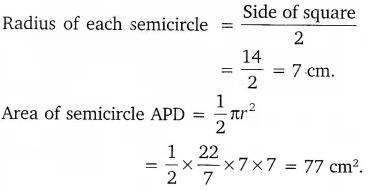Similarly, area of semi-circle BPC = 77 cm2
∴ Total area of two semi-circle = (77 + 77) cm2
= 154 cm2
= Area of the square ABCD – Area of semi-circles
= (196 – 154) cm2 = 42 cm2

Question 4.
Find the area of the shaded region in the figure, where a circular arc of radius 6 cm has been drawn with vertex O of an equilateral triangle OAB of side 12 cm as centre.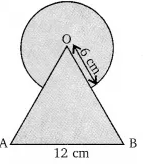Solution:
Area of the equilateral triangle OAB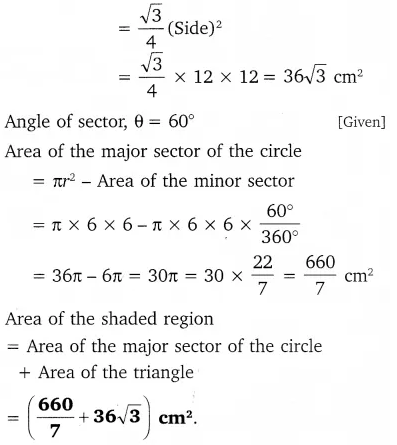Question 5.
From each corner of a square of side 4 cm a quadrant of a circle of radius 1 cm is cut and also a circle of diameter 2 cm is cut as shown in the figure. Find the area of the remaining portion of the square.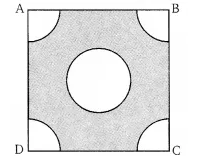Solution:
ABCD of the square ABCD = (4)2 cm2 = 16 cm2.
Area of circel inside the square = πr2
= π × (1)2 = π cm2.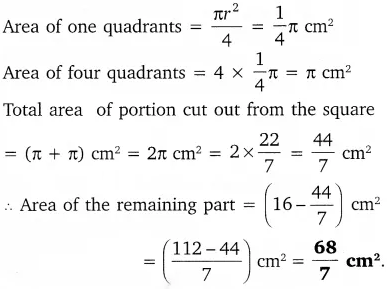Question 6.
In a circular table cover of (he radius 32 cm, a design Is formed leaving an equilìtcral triangle ABC in the middle as shown in the figure. Find the area of the design (shaded region).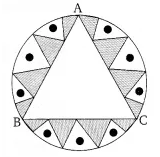Solution:
Let O be the centre of a circular table and ABC be the equilateral triangle.
Then we draw OD ⊥ BC
In ∆OBD, we have: cos 60° = $$\frac{\mathrm{OD}}{\mathrm{OB}}$$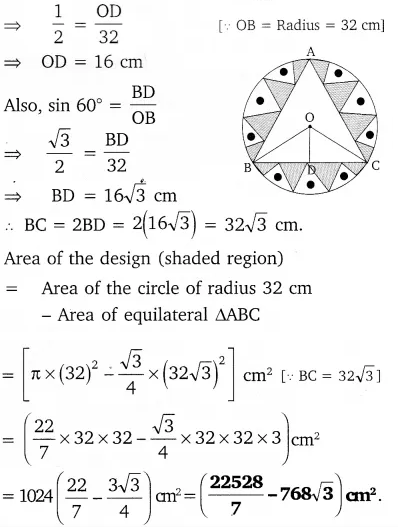Question 7.
In the figure, ABCD is a square of side 14 cm. With centres A, B, C and D, four circles are drawn such that each circle touch externally two of the remaining three circles. Find the area of the shaded region.
Solution:
Each side of the square ABCD = 14 cm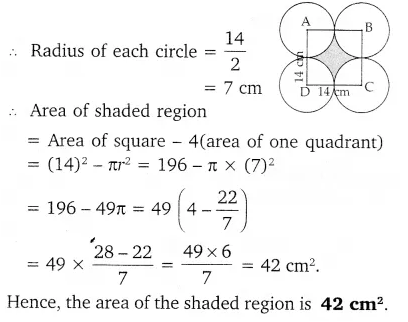Question 8.
The given figure depicts a racing track whose left and right ends are semicircular. The distance between the two inner parallel line segments is 60 m and they are each 106 m long. If the track is 10 m wide, find: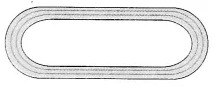(i) the distance around the track along its inner edge.
(ii) the area of the track.
Solution:
The distance around the track along the inner edge = Perimeter of GHI + Perimeter JKL + GL + IJ
= (π × 30 + π × 30 + 106 + 106)
= (60π + 212) m
= (60 × $$\frac{22}{7}$$ + 212)m = $$\frac{2804}{7}$$ m.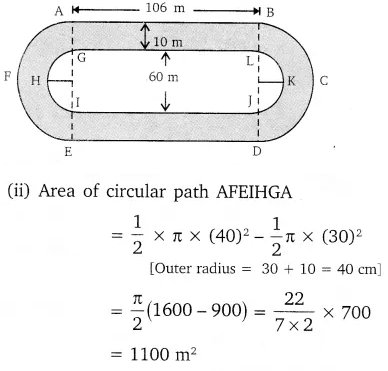Similarly, area of another circular path
BCDJKLB = 1100 m2
Area of rectangular path (ABLG) = 106 × 10
= 1060 m2
Similarly, area of another rectangular path
IJDE = 1060 m2
Total area of the track
= (1100 + 1100 + 1060 + 1060) m2
= (2200 + 2120) m2 = 4320 m2.Question 9.
In the given figure, AB and CD are two diameters of a circle (with centre O) perpendicular to each other and OD is the diameter of the smaller circle. If OA = 7 cm, find the area of the shaded region.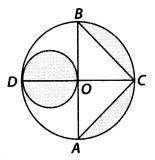Solution:
O is the centre of the circle and OA = 7 cm
⇒ AB = 2OA = 2 × 7 cm = 14 cm
∵ AB and CD are perpendicular to each other.
⇒ OC ⊥ AB and OC = OA = 7 cm
Area of ∆ABC = $$\frac{1}{2}$$ × AB × OC
= $$\frac{1}{2}$$ × 14 cm × 7cm = 49 cm2
Again, OD = OA = 7 cm
∴ Radius of the small circle = $$\frac{1}{2}$$ (OD)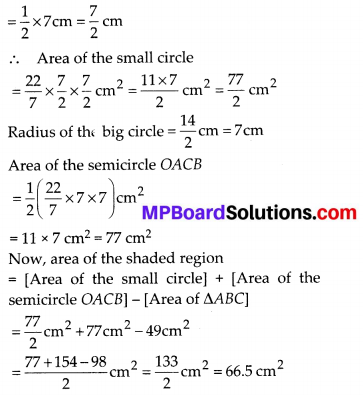Question 10.
The area of an equilateral triangle ABC is 17320.5 cm2. With each vertex of the triangle as centre, a circle is drawn with radius equal to half the length of the side of the triangle (see figure). Find the area of the shaded region. (Use π = 3.14 and $$\sqrt{3}$$ = 1.73205).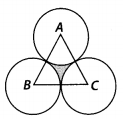Solution:
Area of ∆ABC = 17320.5 cm2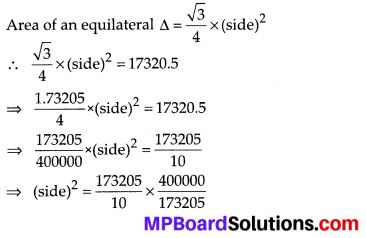⇒ (side)2 = 40000
⇒ (side)2 = (200)2
⇒ side = 200 cm
∴ Radius of each circle = $$\frac{200}{2}$$ cm = 100 cm
Since, each angle of an equilateral triangle is 60°,
∠A = ∠B = ∠C = 60°
Area of a sector having angle of sector (θ), as 60° and radius (r) = 100 cm = $$\frac{\theta}{360^{\circ}}$$ × πr2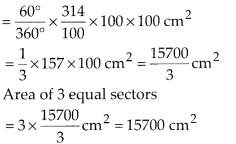Now, area of the shaded region = [Area of the equilateral triangle ABC] – [Area of 3 equal sectors]
= 17320.5 cm2 – 15700 cm2 = 1620.5 cm2

Question 11.
On a square handkerchief, nine circular designs each of radius 7 cm are made (see figure). Find the area of the remaining portion of the handkerchief.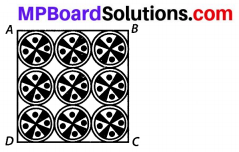Solution:
∵ The circles touch each other.
∴ The side of the square ABCD
= 3 × diameter of a circle = 3 × (2 × radius of a circle) = 3 × (2 × 7) cm = 42 cm
⇒ Area of the square ABCD = 42 × 42 cm2
= 1764 cm2
Now, area of one circle
= πr2 = $$\frac{22}{7}$$ × 7 × 7 cm2 = 154cm2
∴ Total area of 9 circles = 9 × 154 cm2 = 1386 cm2
∴ Area of the remaining portion of the handkerchief = (1764 – 1386) cm2 = 378 cm2Question 12.
In the given figure, OACB is a quadrant of a circle with centre O and radius 3.5 cm. If OD = 2 cm, find the area of the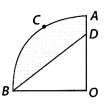Solution:
(i) Here, radius (r) = 3.5 cm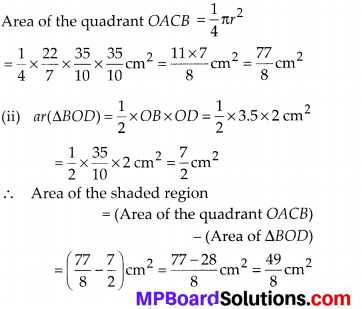Question 13.
In the given figure, a square OABC is inscribed in a quadrant OPBQuestion If OA = 20 cm, find the area of the shaded region (Use π = 3.14)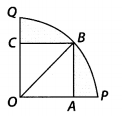Solution:
OABC is a square such that its side
OA = 20 cm
∴ OB2 = OA2 + AB2
⇒ OB2 = 202 + 202 = 400 + 400 = 800
⇒ OB = $$\sqrt{800}=20 \sqrt{2}$$
⇒ Radius of the quadrant (r) = $$20 \sqrt{2}$$ cm
Now, area of the quadrant OPBQ = $$\frac{1}{4}$$ πr2
= $$\frac{1}{4} \times \frac{314}{100}$$ × 800 cm2 = 314 × 2 = 628 cm2
Area of the square OABC = 20 × 20 cm2 = 400 cm2
∴ Area of the shaded region
= 628 cm2 – 400 cm2 = 228 cm2

Question 14.
AB and CD are respectively arcs of two concentric circles of radii 21 cm and 7 cm and centre O (see figure). If ∠AOB = 30°, find the area of the shaded region.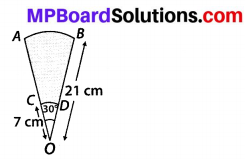Solution:
∵ Radius of bigger circle R = 21 cm and sector angle θ = 30°
∴ Area of the sector OAB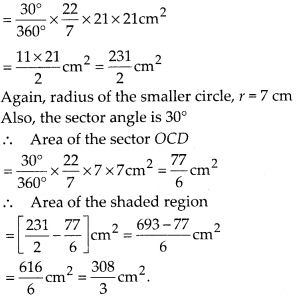Question 15.
In the given figure, ABC is a quadrant of a circle of radius 14 cm and a semicircle is drawn with BC as diameter. Find the area of the shaded region.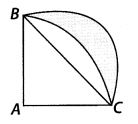Solution:
Since, BC is a diameter in a semi-circle.
∴ ∠BAC = θ = 90°
Area of the semicircle ABPC
= [$$\frac{90^{\circ}}{360^{\circ}} \times \frac{22}{7}$$ × 14 × 14] cm2
= 22 × 7 cm2 = 154 cm2
Area of right ∆ABC
= $$\frac{1}{2}$$ × 14 × 14 cm2 = 98 cm2
⇒ Area of segment BPC = 154 cm2 – 98 cm2
= 56 cm2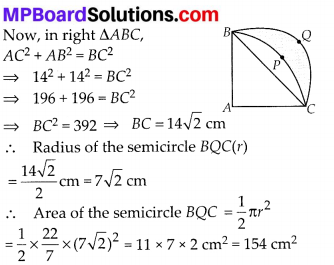Now, area of the shaded region = [Area of semicircle BQC] – [Area of segment BRC]
= 154 cm2 – 56 cm2 = 98 cm2

Question 16.
Calculate the area of the designed region in the given figure, common between the two quadrants of circles of radius 8 cm each.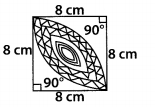Solution:
Side of the square = 8 cm
Area of the square ABCD = 8 × 8 cm2 = 64 cm2